CBSE Class 10 Sample Paper for 2019 Boards

Class 10
Solutions of Sample Papers for Class 10 Boards

Question 15

Places A and B are 80 km apart from each other on a highway. A car starts from A and another from B at the same time. If they move in same direction they meet in 8 hours and if they move towards each other they meet in 1 hour 20 minutes. Find the speed of cars.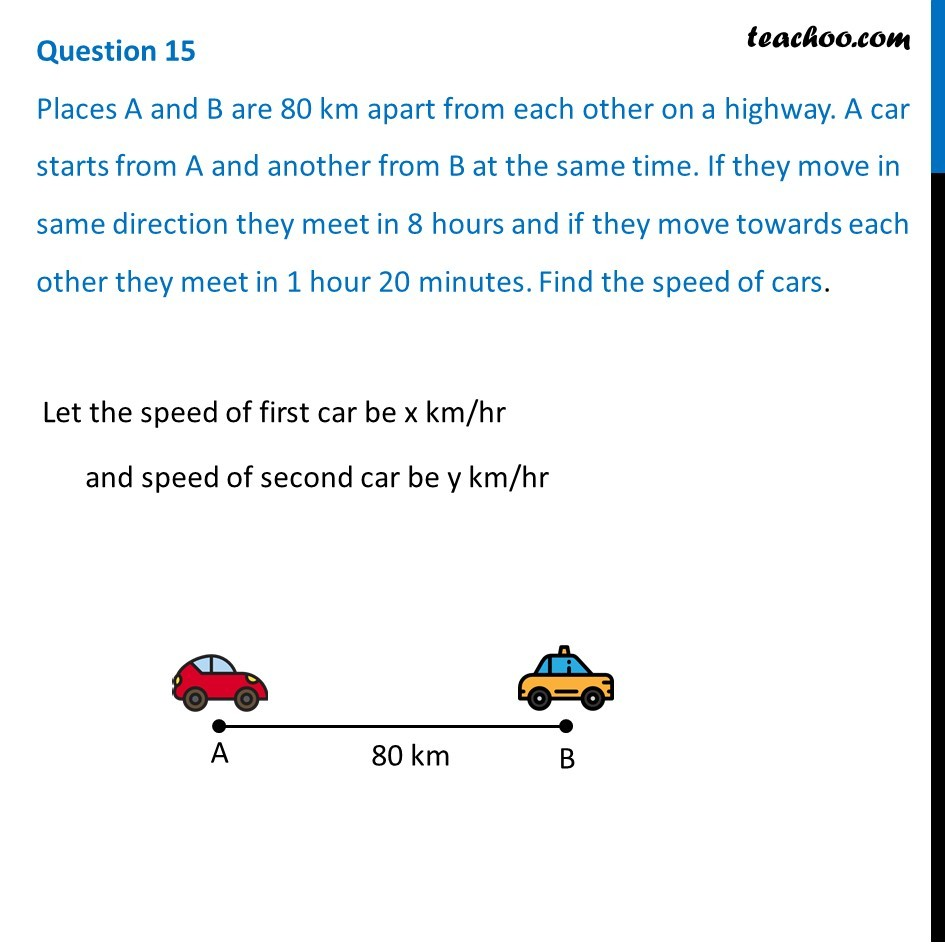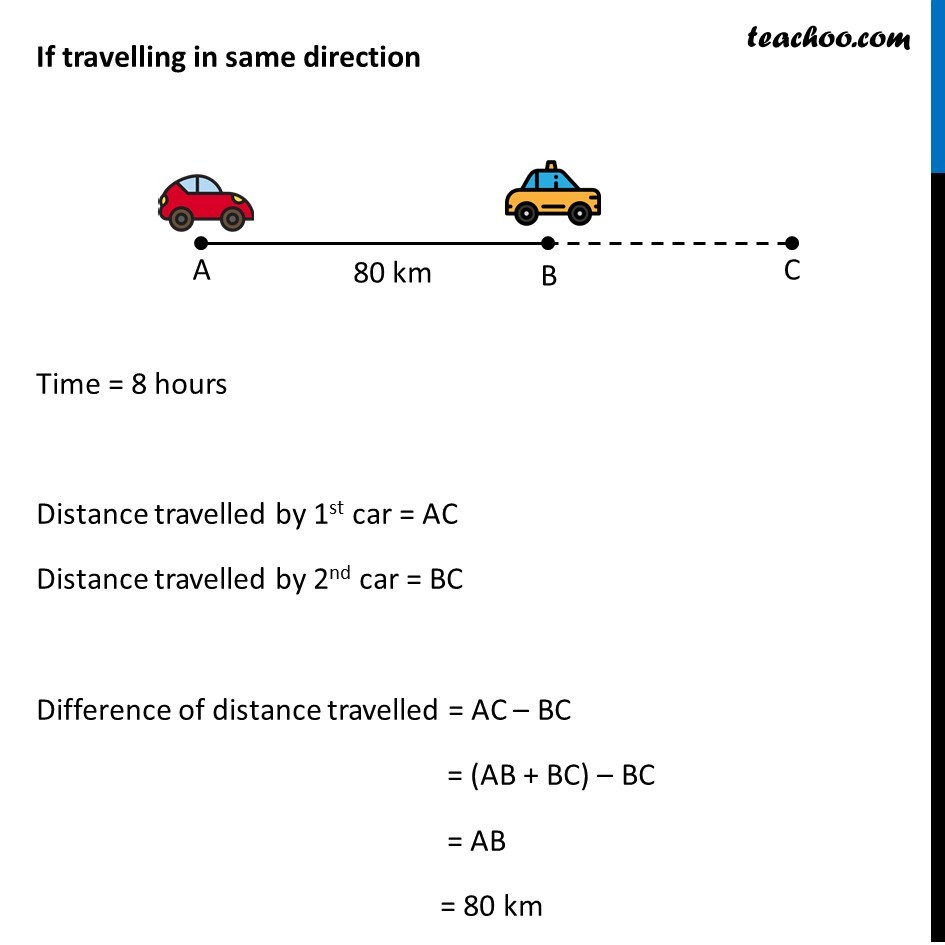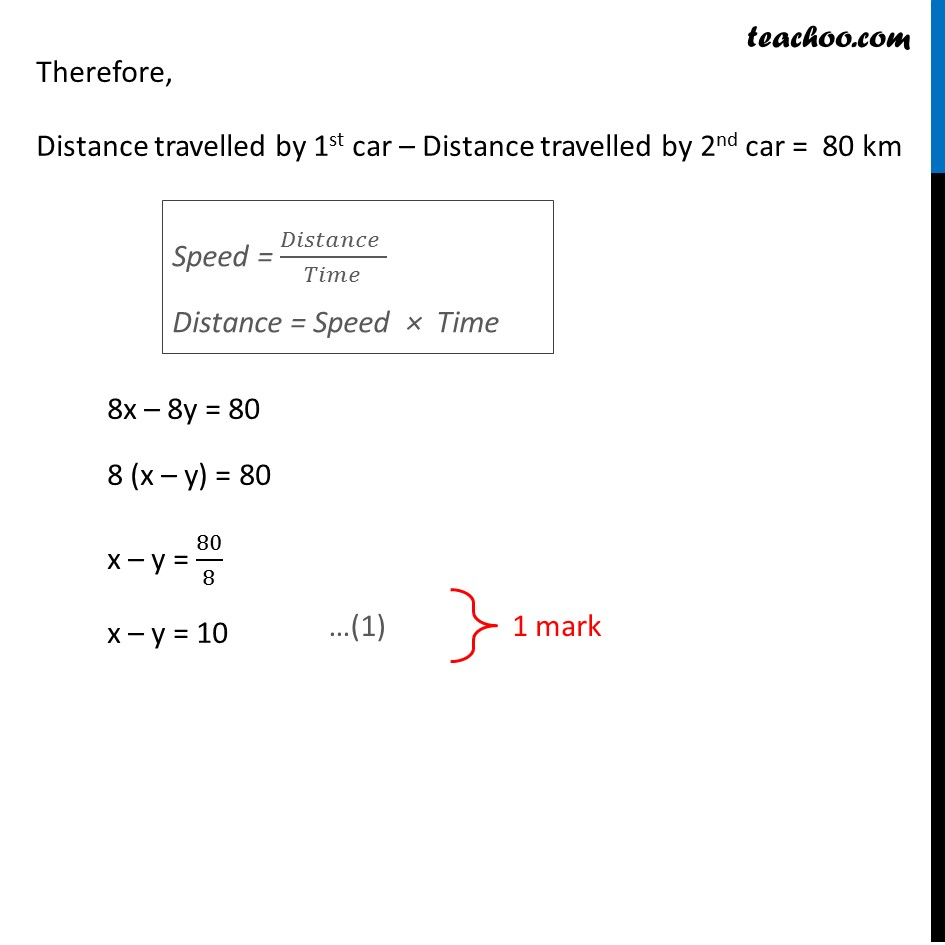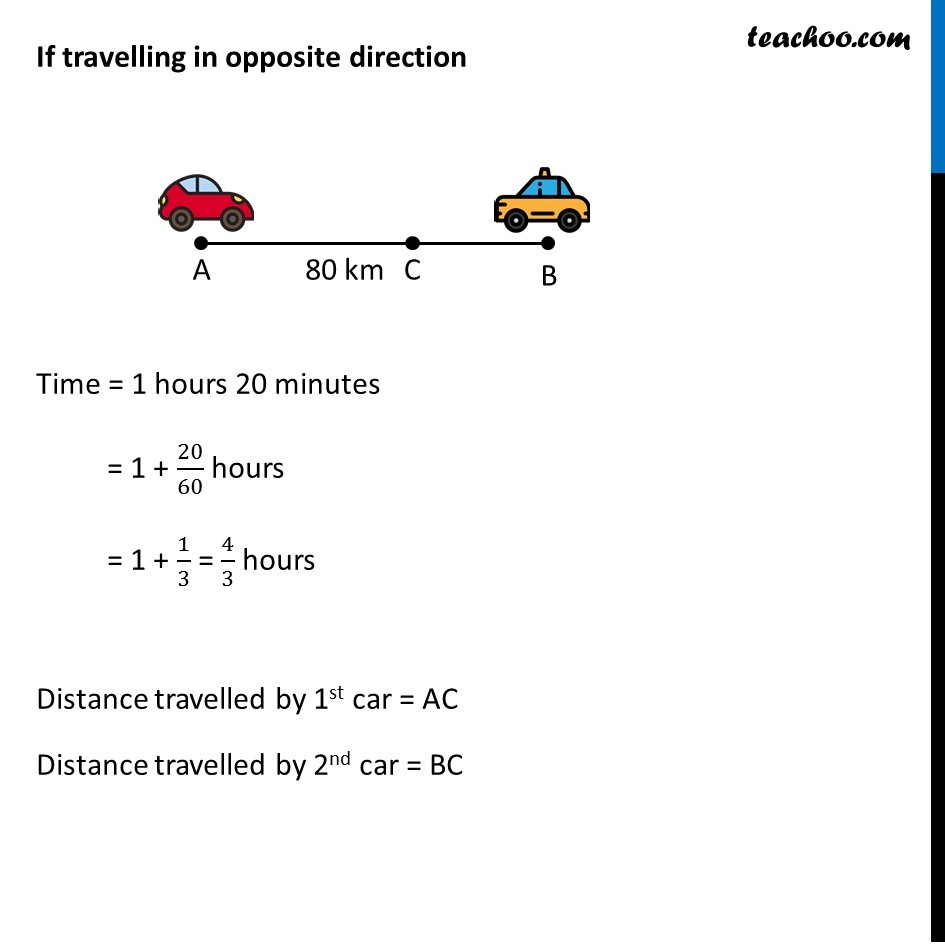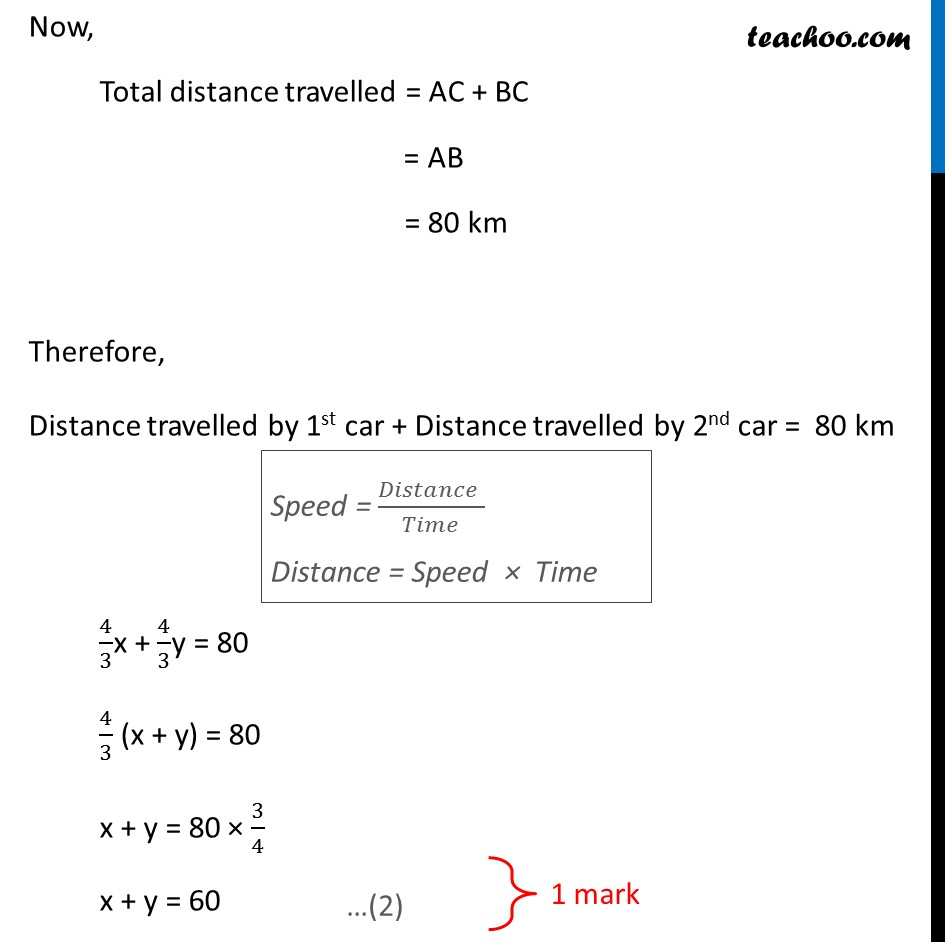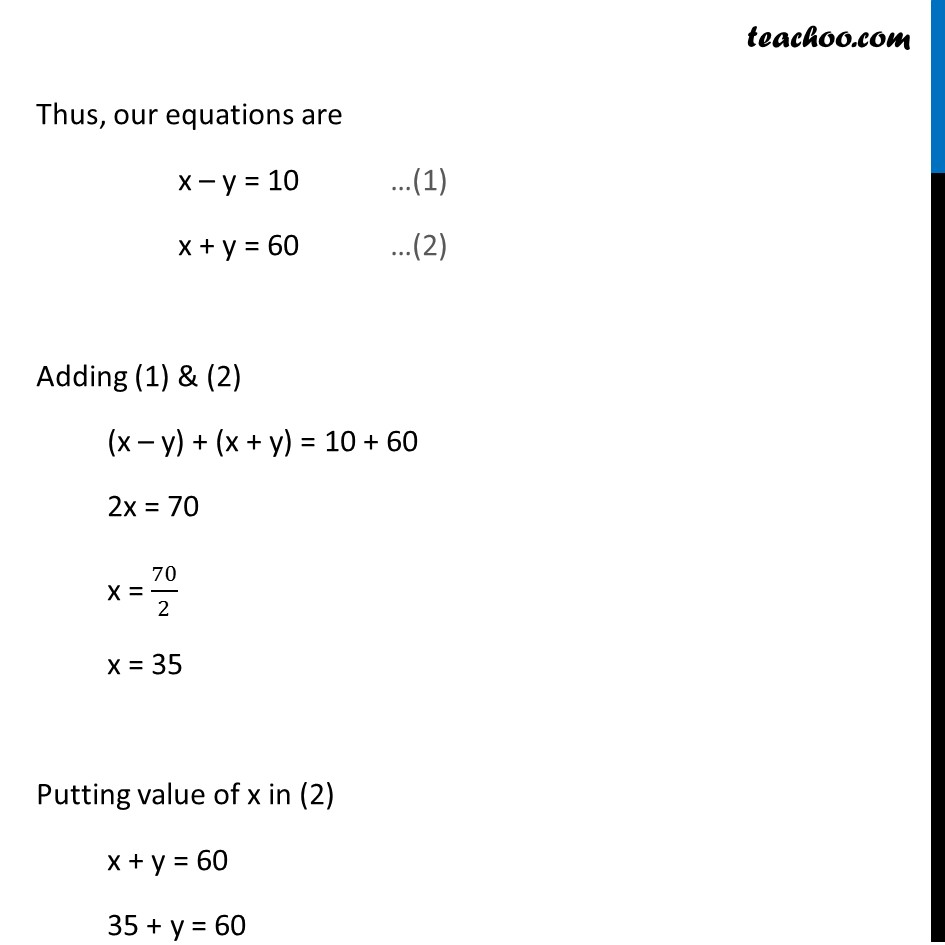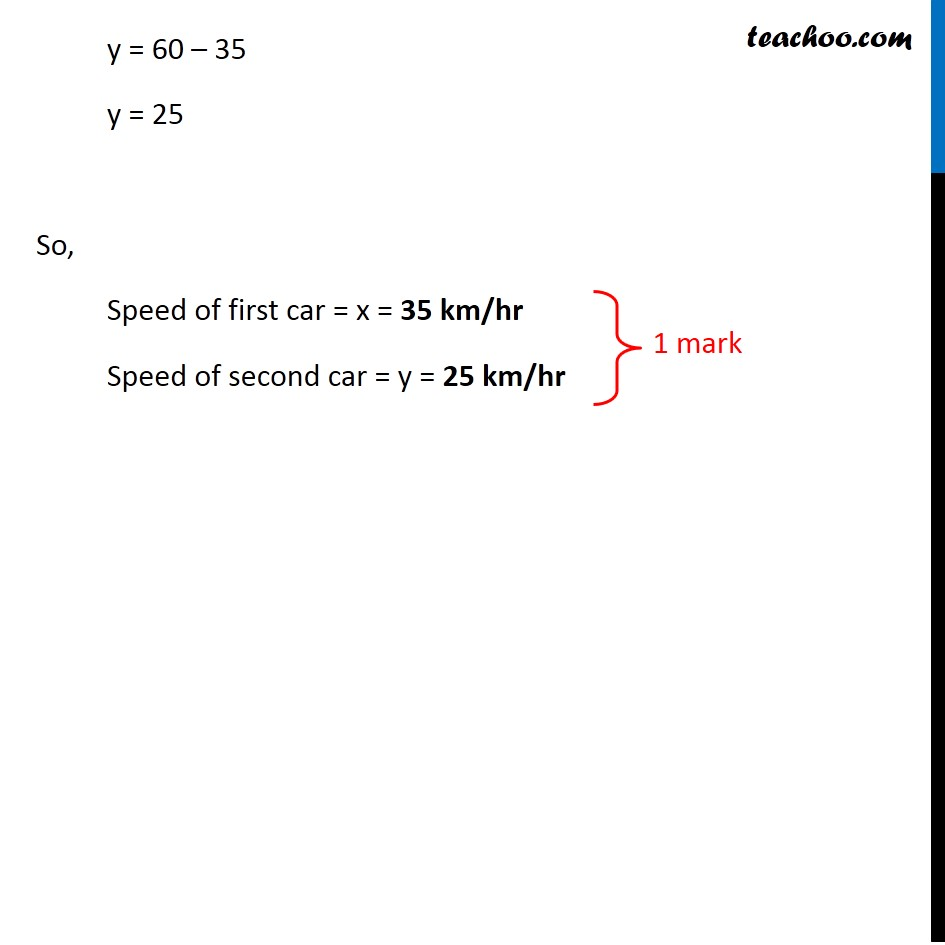Learn in your speed, with individual attention - Teachoo Maths 1-on-1 Class

### Transcript

Question 15 Places A and B are 80 km apart from each other on a highway. A car starts from A and another from B at the same time. If they move in same direction they meet in 8 hours and if they move towards each other they meet in 1 hour 20 minutes. Find the speed of cars. Let the speed of first car be x km/hr and speed of second car be y km/hr If travelling in same direction Time = 8 hours Distance travelled by 1st car = AC Distance travelled by 2nd car = BC Difference of distance travelled = AC – BC = (AB + BC) – BC = AB = 80 km Therefore, Distance travelled by 1st car – Distance travelled by 2nd car = 80 km Speed = (𝐷𝑖𝑠𝑡𝑎𝑛𝑐𝑒 )/𝑇𝑖𝑚𝑒 Distance = Speed × Time 8x – 8y = 80 8 (x – y) = 80 x – y = 80/8 x – y = 10 …(1) 1 mark If travelling in opposite direction Time = 1 hours 20 minutes = 1 + 20/60 hours = 1 + 1/3 = 4/3 hours Distance travelled by 1st car = AC Distance travelled by 2nd car = BC Now, Total distance travelled = AC + BC = AB = 80 km Therefore, Distance travelled by 1st car + Distance travelled by 2nd car = 80 km Speed = (𝐷𝑖𝑠𝑡𝑎𝑛𝑐𝑒 )/𝑇𝑖𝑚𝑒 Distance = Speed × Time 4/3x + 4/3y = 80 4/3 (x + y) = 80 x + y = 80 × 3/4 x + y = 60 …(2) 1 mark Thus, our equations are x – y = 10 …(1) x + y = 60 …(2) Adding (1) & (2) (x – y) + (x + y) = 10 + 60 2x = 70 x = 70/2 x = 35 Putting value of x in (2) x + y = 60 35 + y = 60 y = 60 – 35 y = 25 So, Speed of first car = x = 35 km/hr Speed of second car = 25 km/hr 1 mark